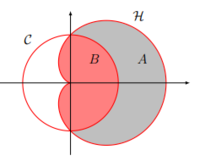# volume integrals in polar coordinates

#### Maxim

##### New member
Hi, in preparations of my calculus exam I have been making exams of the previous years, but I've tried the next question twice but fail to get the right answer. the problem goes as follows:circle c: x² + y² = 1
the heart shape has a corresponding equation of (x-1/2)² + y² -sqrt(x²+y²) = 1/4

a) transform both functions to polar coordinates.
b) find the volume of the body enclosed vertically by the planes z=0 and z=4 and horizontally enclosed by the edge of the gray colored area A.
c) find the volume of the body enclosed vertically by the planes z=0 and z=4 and horizontally enclosed by the edge of the red colored area B.

For part a I get r² = 1 and r²cos²a - r*cos a +r²sin²a - r = 0 with a being the angle

for part b i'm not even sure I know which area he wants me to calculate. I think area B but i'm not sure. but when I try to integrate ( Z from 0-4, r from 0 - sqrt1 and a from 0 to 2pi) I get a volume of zero. I assume the a needs to be a different value but I'm not sure which that would be. the supposed right answer is 8+pi

for part C i would calculate the overall volume of the cylinder C and subtract the answer from B but I can't really verify without solving B first.

Many thanks

-Maxim

#### MarkFL

##### Super Moderator
Staff member
When I convert the rectangular equations to polar, I get:

$$\displaystyle r_1=1$$

$$\displaystyle r_2=\cos(\theta)+1$$

This is what you would get upon simplifying.

Now, the gray area $$A_1$$ can be found by:

$$\displaystyle A_1=\frac{1}{2}\int_{-\frac{\pi}{2}}^{\frac{\pi}{2}}r_2^2-r_1^2\,d\theta$$

Please confirm that you get:

$$\displaystyle A_1=\frac{8+\pi}{4}$$

And then we can continue.#### Maxim

##### New member
thank you for your swift reply.when calculating the integral you gave I do indeed get the correct answer after integrating to Z. I also get why you use a as pi/2 , but where does the 1/2 in front of the integral come from?

#### MarkFL

##### Super Moderator
Staff member
Basically, when doing integrals in polar coordinates, we are using circular sectors (rather than the rectangles we use in rectangular coordinates), and the area $$A$$ of a circular sector is given by:

$$\displaystyle A=\frac{1}{2}r^2\theta$$

Thus:

$$\displaystyle dA=\frac{1}{2}r^2\,d\theta$$

#### Maxim

##### New member
ah i see thank you very much i don't have much experience in dealing with polar coordinates so i didn't see the link

•MarkFL

#### MarkFL

##### Super Moderator
Staff member
To find area B, I would observe that we have a semicircle to the right of the vertical axis, and the area in quadrants 2 and 3 are equivalent, so I would write:

$$\displaystyle A_2=\frac{\pi}{2}+2\cdot\frac{1}{2}\int_{\frac{\pi}{2}}^{\pi} r_2^2\,d\theta$$

You should confirm that:

$$\displaystyle A_2=\frac{5\pi-8}{4}$$